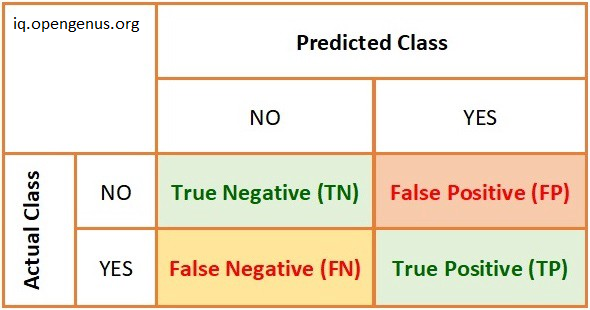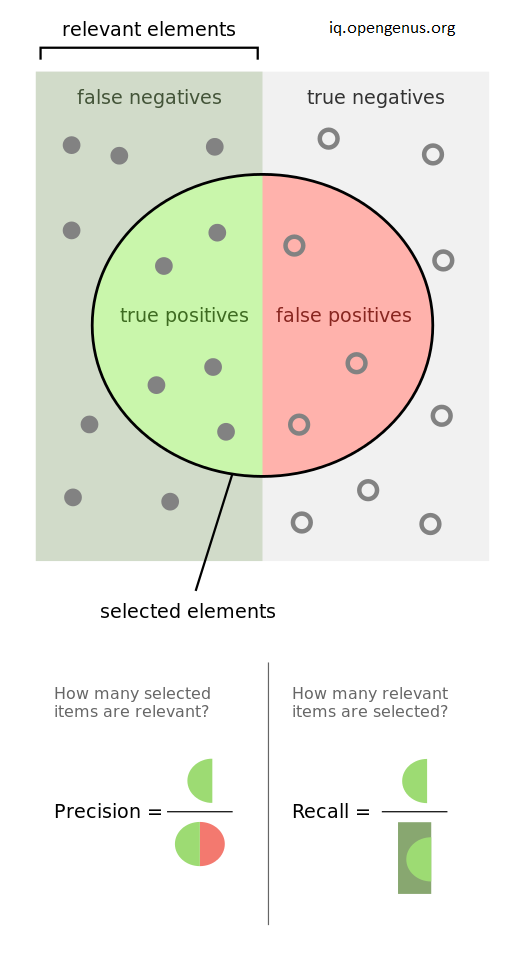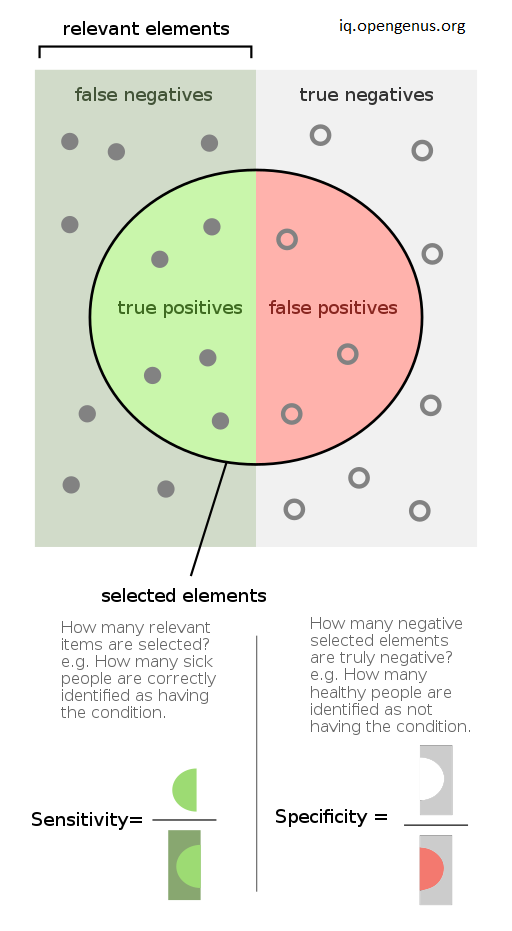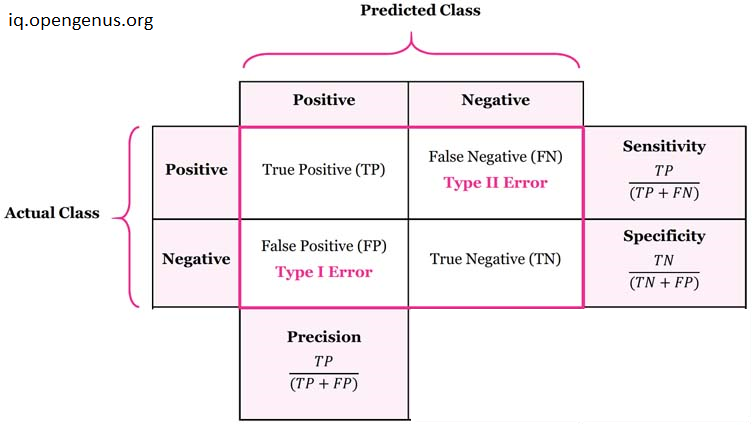×

Search anything:

# Precision, Recall, Sensitivity and Specificity

#### Machine Learning (ML)In this article, we have explained 4 core concepts which are used to evaluate accuracy of techniques namely Precision, Recall, Sensitivity and Specificity. We have explained this with examples.

1. Introduction with an example
2. Confusion Matrix
3. Precision
4. Recall or Sensitivity
5. Specificity
6. How to remember ?
7. Let us have a small test !

Prerequisites:

In order for you to truly understand the differences between each performance metric, I will be using a basic example.

# Introduction with an example

All of the workers at an industry are undergoing a machine learning, primary diabetes scan.

The majority of these tasks are of classification. This is especially true in binary classification. The output is always Boolean, indicating it is either True or False.

The output is either Diabetic (+ve or True) or healthy (-ve or False).

There are only four possible outcomes for any worker X.

• True positive (TP): Prediction is +ve and X is diabetic, Hit, this is what we desire.
• True negative (TN): Prediction is -ve and X is healthy, Correct Rejection, this is what we desire too.
• False positive (FP): Prediction is +ve and X is healthy, false alarm, bad, Over-Estimation (Type I error).
• False negative (FN): Prediction is -ve and X is diabetic, miss, the worst,Under-Estimation (Type II error).

# Confusion Matrix

Confusion matrix is an easy-to-understand cross-tab of actual and predicted class values. It contains the total number of data points that fall in each category.### So, How we determine that our Classification task in good ?

The four categories enable us in determining the classification's quality ->

1. Precision
2. Recall
3. Sensitivity
4. Specificity# Precision

Precision is the Ratio of true positives to total predicted positives.

Precision = TP / (TP + FP)

Numerator: +ve diabetes workers.
Denominator: Our algorithm recognised all +ve diabetes workers, whether or not they are diabetic in reality.

## What Precision tells us ?

How many of individuals we forecasted as diabetes are truly diabetic? Precision offers us the answer to this question.

Precision Used Important
When When the occurrence of false positives is Unacceptable. When we want to be more confident of your predicted positives.
Example Spam emails. You’d rather have some spam emails in your inbox than miss out some regular emails that were incorrectly sent to your spam box.

# Recall or Sensitivity

Recall or Sensitivity is the Ratio of true positives to total (actual) positives in the data.

Recall and Sensitivity are one and the same.

Recall = TP / (TP + FN)

Numerator: +ve labeled diabetic people.
Denominator: All diabetic workers, whether or not our algorithm has identified them.

## What Recall or Sensitivity tells us ?

Recall answer to this question, Of all the workers who are diabetic, how many of them did we properly predict?

Recall Used Important
When When the occurrence of false negatives is unacceptable. When we want to identifying the positives is crucial.
Example When predicting financial default or a deadly disease or Security checks in airports. More false positives than fewer false negatives.

# SpecificitySpecificity is the Ratio of true negatives to total negatives in the data.
Specificity is the correctly -ve labeled by the program to all who are healthy in reality.

Specificity = TN / (TN + FP)

Numerator: -ve labeled healthy worker.
Denominator: All workers who are healthy in actuality, regardless of whether they are classed as +ve or -ve.

## What Specificity tells us ?

How many people who are healthy did we accurately predict? Specificity offers us the answer to this question.

Recall Used Important
When When you don't want to frighten people with misleading information. When you wish to cover all areas where negative categorization is a top priority.
Example A drug test in which all people who test positive will immediately go to jail or Diagnosing for a health condition before treatment.

# How to remember ?

Many cannot remember the difference between sensitivity, specificity, precision, accuracy, and recall, despite having encountered these phrases multiple times. These are very basic terms, but the names are unintuitive, thus many keep getting them mixed up. What's a decent approach to think about these ideas such that the names make sense?

For precision and recall, each is the true positive (TP) as the numerator divided by a different denominator.

Precision and Recall: focus on True Positives (TP).

• Precision: TP / Predicted positive
• Recall: TP / Real positive

Sensitivity and Specificity: focus on Correct Predictions.
There is one concept viz., SNIP SPIN.

• SNIP (SeNsitivity Is Positive): TP / (TP + FN)
• SPIN (SPecificity Is Negative): TN / (TN + FP)

SNIP refers to Sensitivity.
SPIN refers to Specificity.

# Let us have a small test !

1. What is the formula of Precision ?

A. TP / (TP + FP)
B. TN / (TN + FP)
C. TP / (TP + FN)
D. TP / TN
E. None of the Above.

Option A is the right answer.

1. Which two performance metric are similar ?

A. Precision and Recall.
B. Recall and Specificity.
C. Recall and Sensitivity.
D. Precision and Sensitivity.
E. None of these.

Option C is the right answer.

1. Which matrix is the cross-tab of actual and predicted class values ?

A. Similarity matrix.
B. Confusion matrix.
C. Diagonal Matrix.
D. Null Matrix.
E. Identity Matrix.

Option B is the right answer.

1. In which case, the occurrence of false negatives is undesirable ?

A. Precision
B. F1-Score
C. Specificity
D. Sensitivity
E. None of these.

Option D is the right answer.

1. Precision and Recall focus on what Outcomes ?

A. TP
B. TN
C. FP
D. None of these.
E. FN

Option A is the right answer.

1. Which metric helps us when Identifying the positives is crucial ?

A. Recall
B. Precision
C. F1-Score
D. None of the these.
E. Sensitivity

Option A and E are the right answer.

# Let us Summarize nowWith this article at OpenGenus, you must have the complete idea of Precision, Recall, Sensitivity and Specificity.#### Abhijeet Rojatkar

Pursuing M. Tech in Power and Energy Systems from NIT, Karnataka | B. Tech in Electrical Engineering from College of Engineering, Pune

Precision, Recall, Sensitivity and Specificity